# PostgreSQL CHECK constraint

## CHECK constraint

The PostgreSQL CHECK constraint controls the value of a column(s) being inserted.

The PostgreSQL provides the CHECK constraint, which allows the user to define a condition, that a value entered into a table, has to satisfy before it can be accepted. The CHECK constraint consists of the keyword CHECK followed by parenthesized conditions. The attempt will be rejected when update or insert column values that will make the condition false.

The CHECK constraint in PostgreSQL can be defined as a separate name.

## SQL

``````CREATE TABLE orders(
ord_no integer,
ord_date date,
item_name character(35),
ord_qty numeric,
ord_amount numeric CHECK (ord_amount>0)
);
```
```

## Constraint data dictionary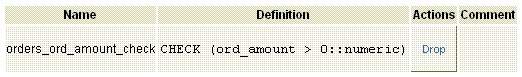Explanation

The above example shows, the table orders have created in which the ord_amount column can not contain any value less than or equal to zero(0).

## SQL

``````CREATE TABLE orders(
ord_no integer,
ord_date date,
item_name character(35),
ord_qty numeric,
ord_amount numeric CONSTRAINT positive_ord_amount CHECK (ord_amount>0)
);
```
```

Output: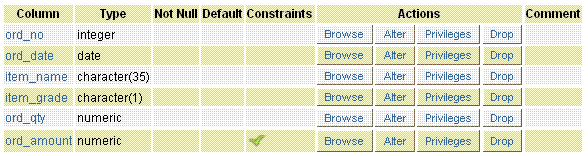## Constraint data dictionary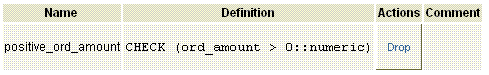Explanation

The above example shows, the table orders have created in which the ord_amount column can not contain any value less than or equal to zero(0). Here, a constraint name has included such a manner that the keyword CONSTRAINT followed by the named constraint positive_ord_amount followed by the expression.

## SQL

``````CREATE TABLE despatch(
des_no integer,
des_date date,
item_name character(35),
ord_qty numeric,
CHECK (ord_qty>0),
des_qty numeric
CHECK (des_qty>0),
CHECK (des_qty>=ord_qty)
);
```
```

Output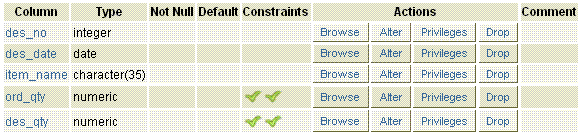## Constraint data dictionary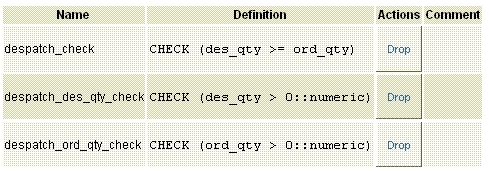Explanation

The above example shows, the table despatch have created in which the ord_qty and des_qty columns can not contain any value less than or equal to zero(0). Here, CHECK constraint refer more than one columns. The first two constraints are column constraint but the third one is table constraint because it is written separately. The column constraint can be written as table constraint but the otherwise not possible.

## SQL

``````CREATE TABLE despatch(
des_no integer,
des_date date,
item_name character(35),
ord_qty numeric,
CHECK (ord_qty>0),
des_qty numeric
CHECK (des_qty>0),
CONSTRAINT valid_qty CHECK(des_qty>=ord_qty)
);
```
```

Output:## Constraint data dictionary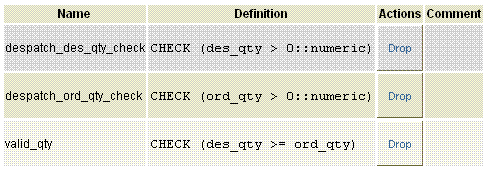Explanation

The above example shows, the table despatch have created in which the ord_qty and des_qty columns can not contain any value less than or equal to zero(0). Here, CHECK constraint refer more than one columns. The first two constraints are column constraint but the third one is table constraint and a name valid_qty have assigned for table constraint.

﻿